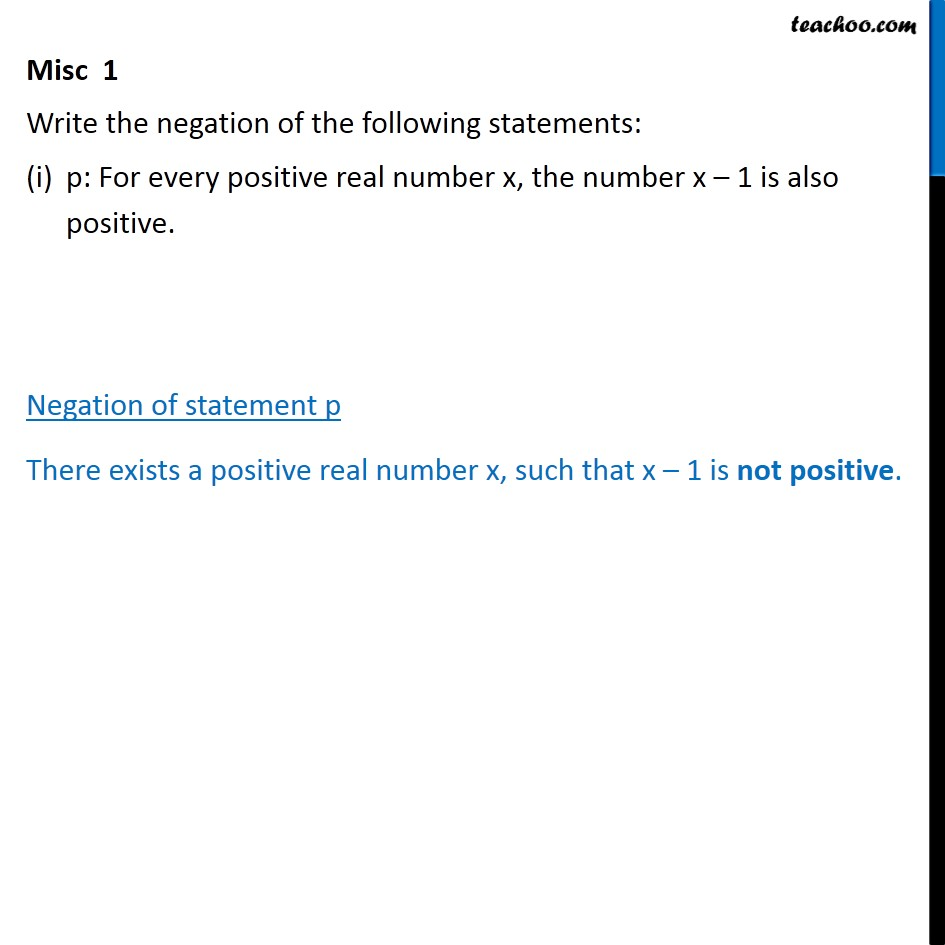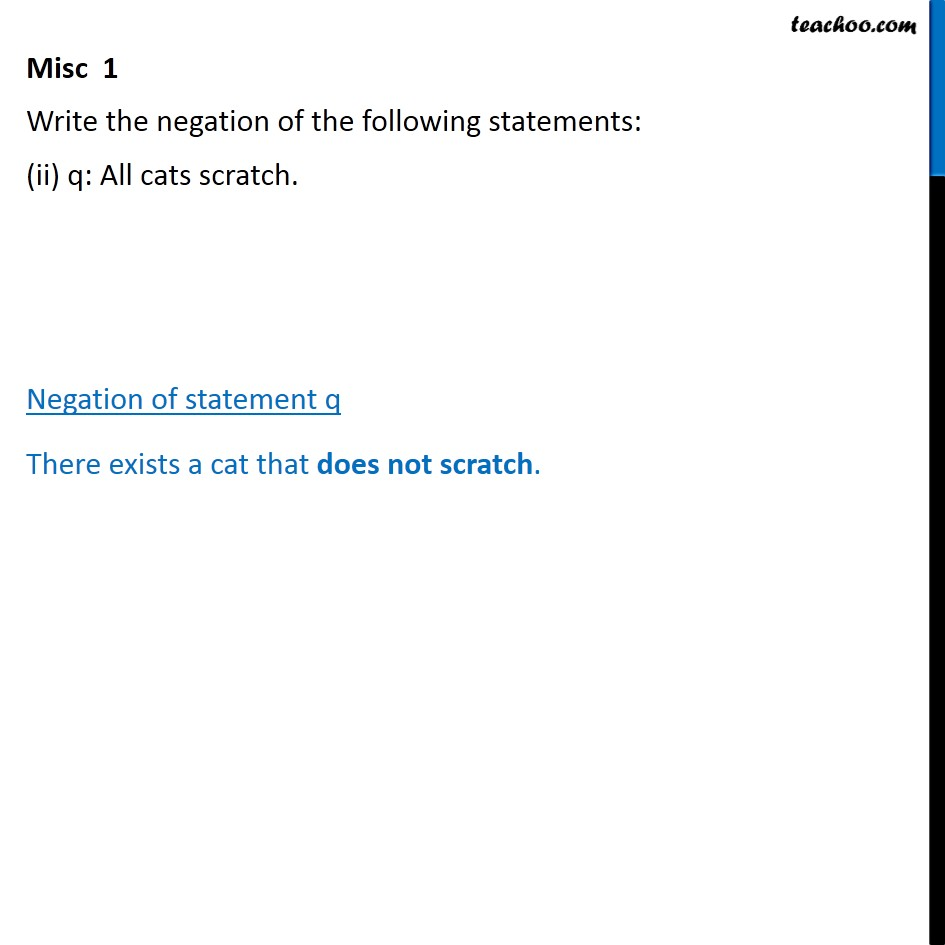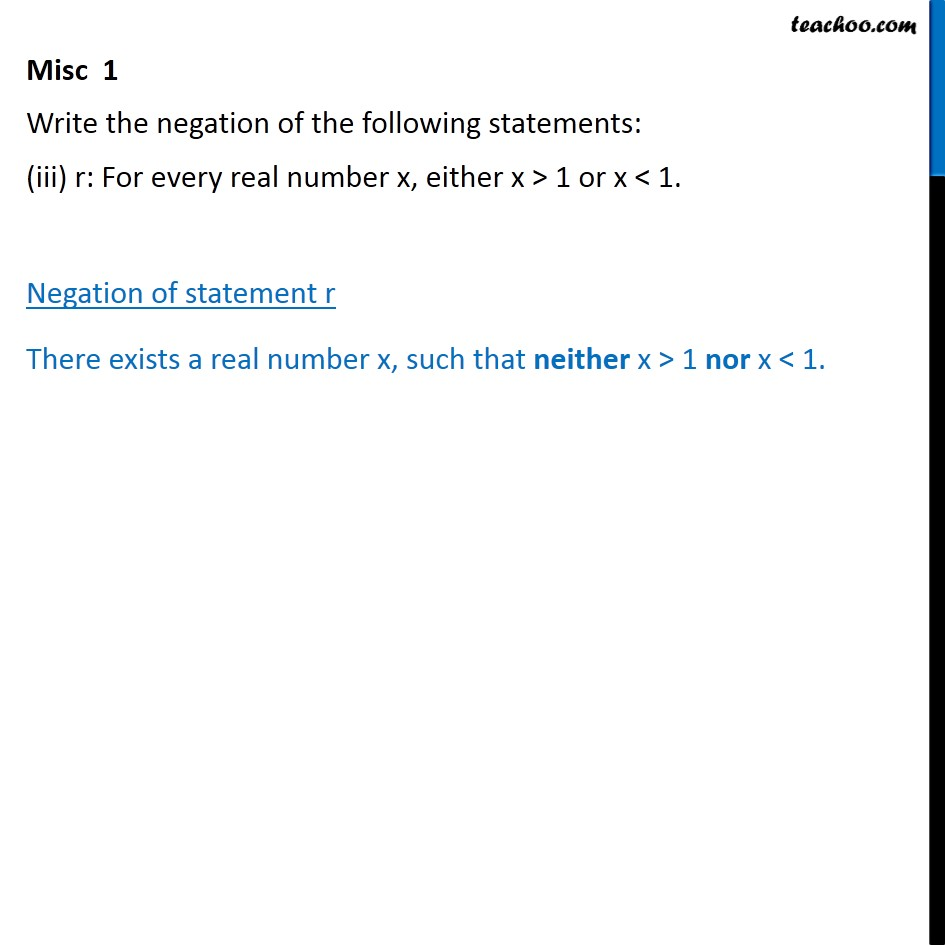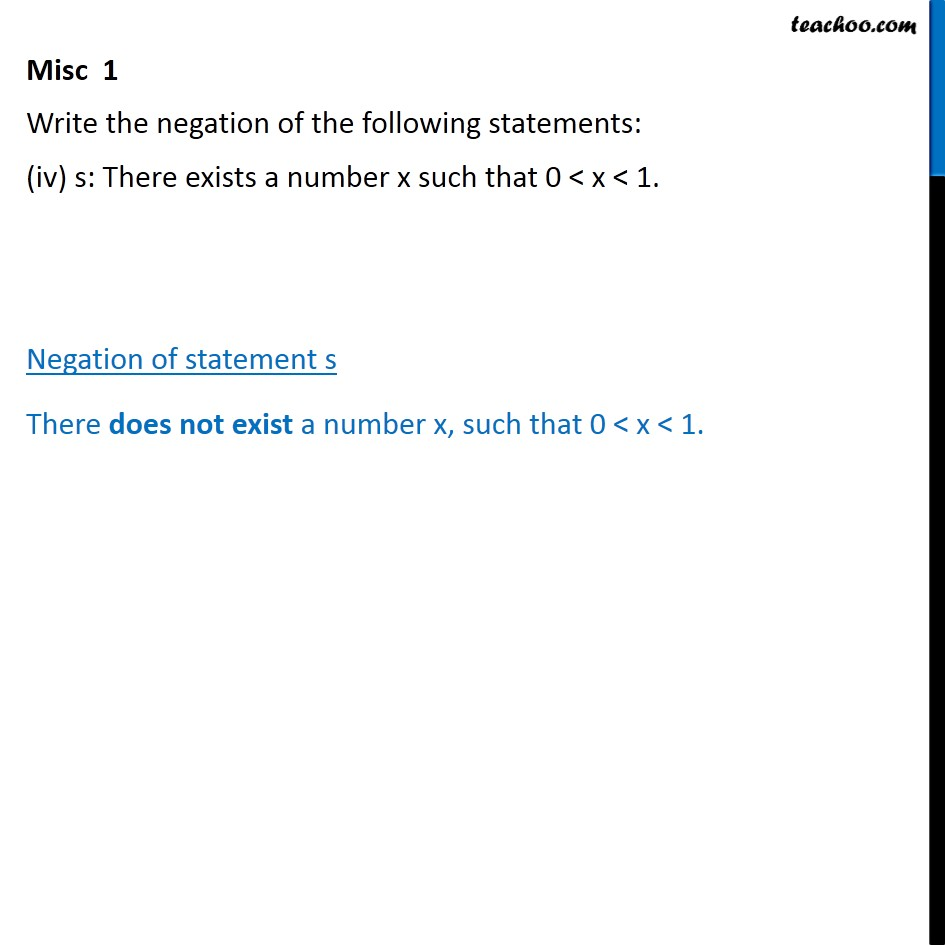Subscribe to our Youtube Channel - https://you.tube/teachoo

1. Chapter 14 Class 11 Mathematical Reasoning
2. Serial order wise
3. Miscellaneous

Transcript

Misc 1 Write the negation of the following statements: p: For every positive real number x, the number x 1 is also positive. Negation of statement p There exists a positive real number x, such that x 1 is not positive. Misc 1 Write the negation of the following statements: (ii) q: All cats scratch. Negation of statement q There exists a cat that does not scratch. Misc 1 Write the negation of the following statements: (iii) r: For every real number x, either x > 1 or x < 1. Negation of statement r There exists a real number x, such that neither x > 1 nor x < 1. Misc 1 Write the negation of the following statements: (iv) s: There exists a number x such that 0 < x < 1. Negation of statement s There does not exist a number x, such that 0 < x < 1.

Miscellaneous